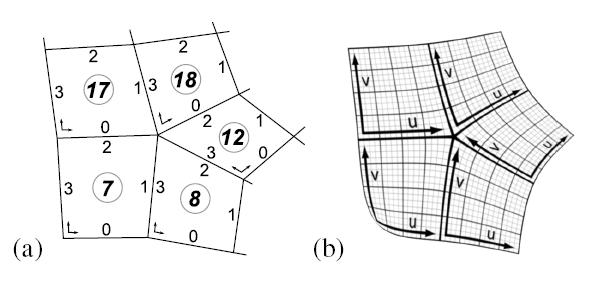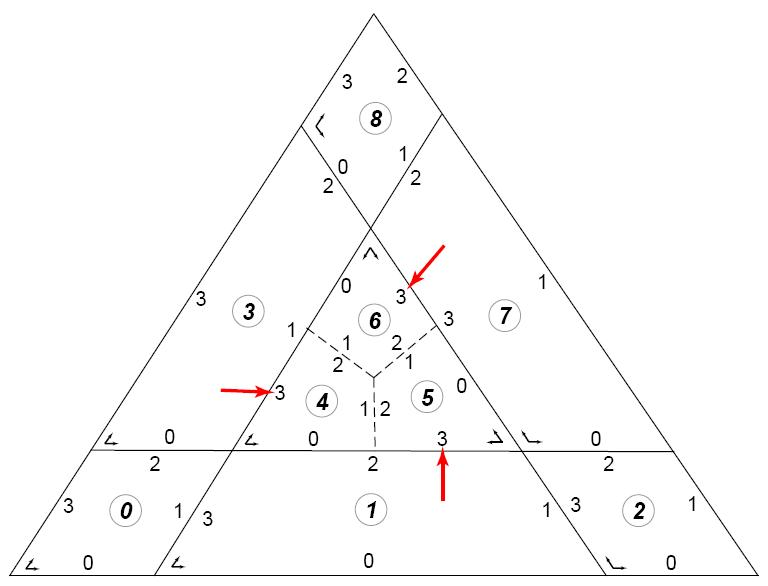Figure 1. a) Portion of a control mesh showing intrinsic faceids and edgeids. b) Corresponding limit surface showing continuous isolines across faces.

In the simplest case, shown in Figure 1, the base mesh for a Ptex texture is an arbitrary quad mesh which is completely and uniquely parameterized using the coordinate <faceid, u, v> where faceid is implied by the position of each face in the mesh description, u and v are the intrinsic quad parameters, and the UV orientation is defined by the vertex ordering (assumed to be <0,0>, <1,0>, <1,1>, <0,1>).

The four edgeids of each quad face are numbered 0 to 3 and follow the vertex ordering. Adjacency data needed for filtering is stored as an array of the four adjacent faceids and edgeids per face. A faceid of -1 indicates that the edge is on a mesh boundary. For instance, face 7 shown in Figure 1 would have adjacency data {adjfaces:<-1,8,17,-1>; adjedges:<x,3,0,x>,x=don't care}.

A more general case with a non-quad face is shown below:Figure 2. Mesh with subfaces resulting from a triangular face (subfaces separated by dashed lines).

For a quad mesh with non-quad faces, the Ptex file contains one texture per subface, as shown in Figure 2. The adjacency data is for the most part the same as for the quad case; each subface has a faceid and references its four adjacent faces and edges within those faces. The only change to the adjacency data is an additional flag in the FaceInfo data structure to indicate whether a face is a subface.

There's one important detail that is specific to quad/non-quad boundaries. When a quad face is adjacent to a non-quad face, it will have two subface neighbors across that edge and it can only refer to one of them in the adjfaces data. In this situation, the first subface encountered in a counter-clockwise (i.e. edgeid order) traversal of the face shall be referenced as the adjacent face. In the example in Figure 2, these cases are indicated by red arrows: face 1 would reference face 5 edge 3 as its neighbor (as opposed to face 4 edge 0), face 7 would reference face 6 edge 3, and face 3 would reference face 4 edge 3. This convention is assumed in the filtering code. A useful feature of this approach is that a vertex can be traversed clockwise by following the adjface indicies without regard to whether a face is a subface or not.

Faces with N edges are mapped with N subfaces and follow the same rules.